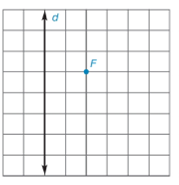Chapter 7.1, Problem 24E### Elementary Geometry for College St...

6th Edition
Daniel C. Alexander + 1 other
ISBN: 9781285195698

#### Solutions

Chapter
Section### Elementary Geometry for College St...

6th Edition
Daniel C. Alexander + 1 other
ISBN: 9781285195698
Textbook Problem
1 views

# For Exercises 21 to 26, use the grid and your compass (as needed) to locate several points on the parabola having the given focus F and directrix d . Then sketch the parabola that is characterized by these points.To determine

To sketch:

The parabola using the below given grid with directrix and focus.

Explanation

Definition:

A parabola is the locus of points that are equidistant from a fixed line and a fixed point not on that line.

The fixed point is known as focus of the parabola and the fixed line is known as directrix of the parabola.

Calculation:

Draw the given directrix d and focus F.

Draw a line parallel to the directrix and passing through the point F.

Draw a perpendicular line to the directrix and passing through F and then locate the mid-point P1.

The point P1 will be the vertex.

Draw a line through P1 parallel to the directrix and locate the point D1 on the directrix.

Locate the points P2, and P3 along the line parallel to the directrix passing through F such that the distance FD1=FP2=FP3

### Still sussing out bartleby?

Check out a sample textbook solution.

See a sample solution

#### The Solution to Your Study Problems

Bartleby provides explanations to thousands of textbook problems written by our experts, many with advanced degrees!

Get Started

#### Let A= Show that (AT)1=(A1)T.

Finite Mathematics for the Managerial, Life, and Social Sciences

#### 37.

Mathematical Applications for the Management, Life, and Social Sciences

#### let f(x) = x3 + 5, g(x) = x2 2, and h(x)= 2x + 4. Find the rule for each function. 2. f g

Applied Calculus for the Managerial, Life, and Social Sciences: A Brief Approach

#### Polar coordinates of the point with rectangular coordinates (5, 5) are: (25, 0)

Study Guide for Stewart's Single Variable Calculus: Early Transcendentals, 8th